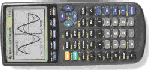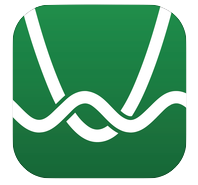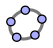# Electronic Graphing

Labs and Lessons by Henri PicciottoI created most of these activities back in the day of the graphing calculator.
If you create a Desmos or GeoGebra version of any of them, e-mail me. I'll link to it and credit you.

These activities span the range from Algebra 1 to Calculus.
Most can be used with any electronic grapher on a calculator, computer or tablet.

## Technology: Friend or Foe?

Some educators, parents, and mathematicians are concerned that the availability of graphing technology can undermine the development of algebraic skills. The activities I linnk to on this page show that used properly, this technology can help develop the mathematical understanding that needs to grow alongside algebraic skills.

The goal of these sample activities is not merely to speed up traditional assignments, or to check answers obtained by other means, or to have access to a magic tool which can answer questions the students do not yet have. The goal is to provoke the sort of discussion and reflection that can deepen students' understanding, a necessary complement to the corresponding skills.

## Other Uses of Electronic Graphing

The lessons below are strongly conceptual, and in my view offer sufficient evidence that electronic graphing is a crucial tool that makes powerful ideas more accessible to more students. However, this is not to say that there are not other valid uses of graphing technology. One important, if controversial, use is to support those students who are less prepared for secondary school math.

Yes, of course, it would be better if all our students had a strong number sense, automated arithmetic skills, and total mastery of fractions. The unfortunate fact is that many start secondary school without those skills and understandings. Luckily, this is no longer a fatal handicap. Today's technology means that mastery of arithmetic is no longer prerequisite for learning algebra.

In fact, the situation has been turned on its head. Many students' number skills will not develop if we continue to slam the door in their face because they lack those skills. If, on the other hand, we support them as they take on the challenge of learning algebra, if we let them continue with high school mathematics, and if we allow them to use the calculator as they see fit along the way, there is a chance for them to grow mathematically in every direction -- including a stronger number sense. I have seen this happen over and over with students at my school, and it breaks my heart to see the in-fact punitive attitude of so many teachers and professors: their well-intentioned ban on calculator use, far from solving the (real!) problems they are concerned about, only serves to perpetuate them.

## Other Technologies

Electronic graphing has been broadly adopted, but is not the only technology relevant to math education. The intellectually impoverished world of "programmed learning" may have a limited role, but it will not improve the quality of instruction in any fundamental way. The use of brief online lectures can be effective if combined with other approaches. However, some technologies do have transformative potential in the mathematics classroom. I am thinking of spreadsheets, computer/calculator algebra systems, probability/statistics software, programming environments, and dynamic geometry. All of them put not just computational power at students' fingertips, but also powerful tools for exploring, discovering, and making. Read more on this topic, and find links to related curriculum, on these pages:
Applets Directory
Tool-Rich Pedagogy
Math: Visual and Interactive!
Why I Use the Interactive Whiteboard

## Make These Designs

Among the activities I have developed, this one is among the most popular. Students enjoy it, and teachers appreciate how it can be used as an engaging introduction or review of an important topic: graphs of linear functions, and the parameters m and b in y=mx+b.

Find the worksheets, an article about the activity, and links to extensions here.

## Stairs

In this activity, students enter a rise and a run, and see the resulting stairs. The challenge can be to connect two given points, to put stairs on a given line, or to put a line on given stairs.

I have used this as a way to review the basics of slope, and to practice such techniques as finding points on a line from the equation, the equation of a line from the coordinates of two points, and the equation of a line from the coordinates of a point and the slope.

Connection: Height and Weight, and Stairs and Squares are two lessons about slope, using stairs, but requiring no technology. They can be found in my book Algebra: Themes, Tools, Concepts (and are available here).

## Off and On / Add Till It's Plaid

Two lessons on the connection between the equation ax+by=c and its graph. They provide a conceptual foundation for two approaches to solving systems of linear equations.

## Which is Greater?

Some students know how to solve linear equations, but don't know what solving an equation means. In my Lab Gear activities (algebra manipulatives), I introduced a genre of exercise called "Which is Greater?" which addresses this situation by asking students to compare two expressions, and thus provides a context for the more specific question "when are the two expressions equal?" (Exercises of this type can now be found in the latest version of the College Preparatory Math Algebra Connections textbook.)

These worksheets "Which is Greater?"(TI-83/84, TI-89) address the same issue through the use of graphing technology. They were inspired by an article by Nurit Zehavi and Giora Mann "Task Design in a CAS Environment: Introducing (In)equations" (a chapter in Computer Algebra Systems in Secondary School Mathematics Education, NCTM, 2003).

The worksheets assume access to ten-sided dice, but can easily be adapted to other approaches: spinners, playing cards, normal six-sided dice, or the random number generator on TI calculators [MATH, PRB, randInt(-9,9)] or other technology. Note that the use of ten-sided dice is slightly misleading, as 0 is twice as likely as the other outcomes in the version using numbers from -9 to 9.

Links to many lessons and activities, to be used in Algebra 1, Algebra 2, and with math teachers, including three paths to the quadratic formula. Look at everything, and decide what you can use! Here's the link.

## Exponential Functions

Two activities to introduce exponential functions and their graphs. Plus an activity for teachers about "all exponential graphs are similar".

## Super-Scientific Notation

An activity to introduce base 10 logarithms building on a solid foundation about the laws of exponents.

## Graphing Square Roots

You may print and duplicate the worksheet (TI-83/84 | TI-89 | GeoGebra)

This lesson takes on a number of student misconceptions, using graphing technology to stimulate discussion and reflection.

Use #1-4 to reinforce the idea that sqrt(x) has a single, positive value, and that it can be less than or greater than x, depending on x. The rest of the lesson leads to the idea that sqrt(x^2)=abs(x). This idea is very difficult for students, in part because they may have done many exercises which involved simplifying radicals with the assumption that the variables are positive, which is of course easier, and perhaps legitimate in the context of the Pythagorean theorem, but certainly misleading as students move on to more advanced classes and need to understand the square root as a function.

The other misconception that is challenged here is the tenacious student belief that sqrt(x^2+4 can be simplified.

Note that the "window" instructions are crucial to the lesson having its full impact: by limiting students to the first quadrant in #5-11, the misconception is brought to the fore; by limiting it to the second quadrant in #12-16, it is challenged; only after that (#17-22) do we look at all four quadrants again, and reach our conceptual destination.

As always, it is essential to complement the worksheet with a full discussion and summary of the important ideas here.

## Computing Geometric Transformations

From my Space course, this is a unit for grade 11 or 12 on using complex numbers, and then matrices, to compute geometric transformations. It involves substantial use of the TI-89 (files). The PDF includes teachers' notes.

## Doctor Dimension

Use graphing technology to help with a discussion of rate of change, continuity, and differentiability in a geometric context: Doctor Dimension.Related pages on this site:
Virtual Grid Paper (not for electronic graphing!)
Applets Directory
Tool-Rich Pedagogy
Math: Visual and Interactive! (using the computer)
Why I teach programming (1997)